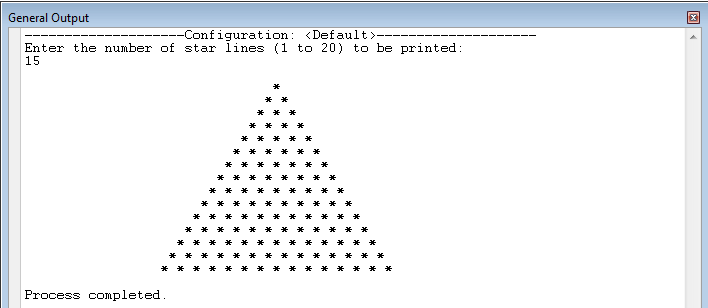# Using Void Methods and Parameters

The following Java program uses Void method s and its Parameters.. I will be using the JCreator IDE in developing the program. To start in this tutorial, first open the JCreator IDE, click new and paste the following code.
`import java.util.*; public class TriangleOfStars{	static Scanner console = new Scanner(System.in); 	public static void main (String[] args)	{		int numberOfLines;		int counter;		int numberOfBlanks; 		System.out.println("Enter the number of star lines " 			+ "(1 to 20) to be printed: ");		numberOfLines = console.nextInt();		System.out.println(); 		while (numberOfLines < 0 || numberOfLines > 20) 		{			System.out.println("The number of star lines "				+ "should between 1 and 20");			System.out.print("Enter the number of star"				+ "lines (1 to 20) to ber printed: ");			numberOfLines = console.nextInt();			System.out.println(); 		}		numberOfBlanks = 30; 		for (counter = 1; counter <= numberOfLines;				counter++)				{					printStars(numberOfBlanks, counter);					numberOfBlanks--;	}	}	public static void printStars(int blanks, int starsInLine)	{		int count; 		for(count =1; count <= blanks; count++)			System.out.print(" ");		for(count =1; count <= starsInLine; count++)			System.out.print(" *");			System.out.println();	}}`
Sample Run:The program works as follows: The statement ` printStars(numberOfBlanks, counter);` in the method `main` is a method call. The method `printStars` has two parameters. Whenever the method executes, it outputs a line of stars with a certain number of blanks before the stars. The number of blanks and the number of stars in the line are passed as parameters to the method `printStars`. The first paramenter, blanks, tells how many blanks to print, second parameter, `starsInLin`, tells how many stars to print in the line. In the method `main`, the user is first asked to specify how many lines of stars to print.
` System.out.println("Enter the number of star lines " 			+ "(1 to 20) to be printed: ");`
The statement
` while (numberOfLines < 0 || numberOfLines > 20) 		{			System.out.println("The number of star lines "				+ "should between 1 and 20");			System.out.print("Enter the number of star"				+ "lines (1 to 20) to ber printed: ");			numberOfLines = console.nextInt();			System.out.println(); `
Ensures that the program prints the triangular grid of stars only if the number of lines between 1 and 20. The statement
` for (counter = 1; counter <= numberOfLines;				counter++)  `
in the method main calls the method `printStars`